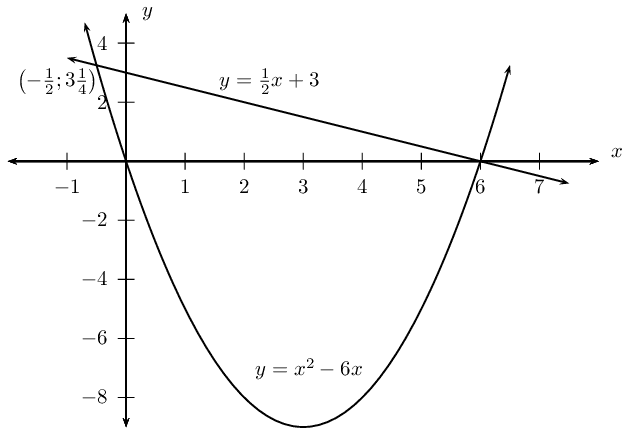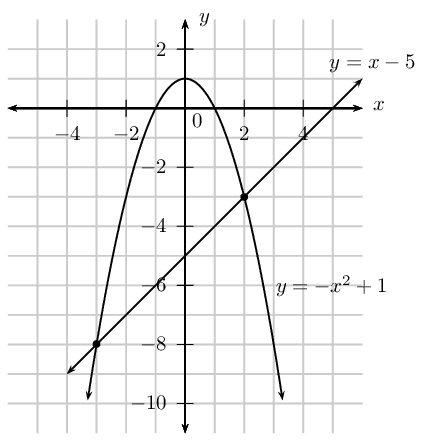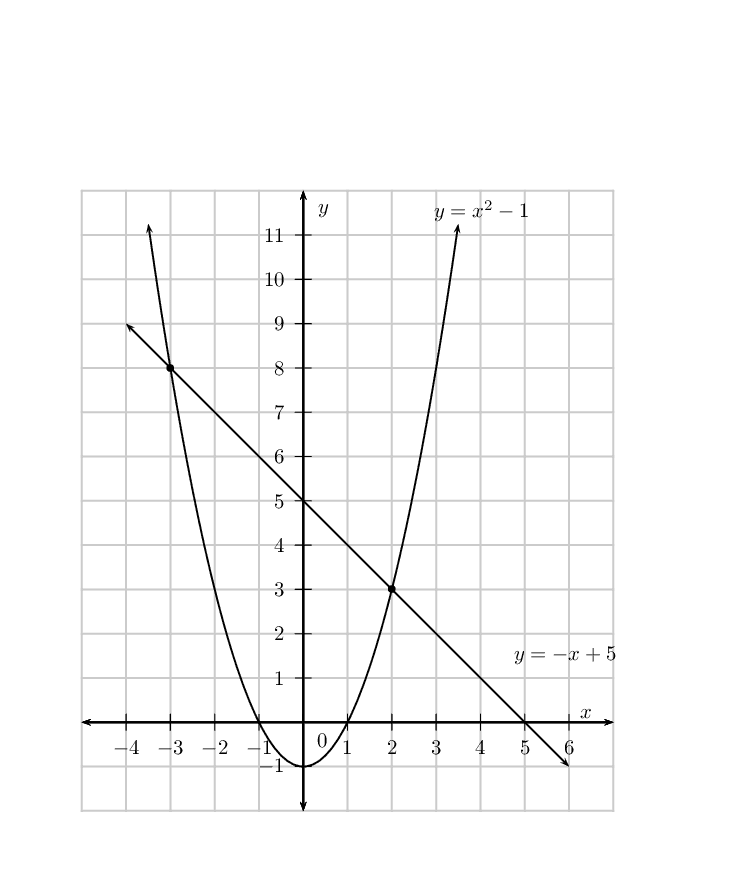We think you are located in United States. Is this correct?

# 2.8 Simultaneous equations

## 2.8 Simultaneous equations (EMBFS)

Simultaneous linear equations can be solved using three different methods: substitution, elimination or using a graph to determine where the two lines intersect. For solving systems of simultaneous equations with linear and non-linear equations, we mostly use the substitution method. Graphical solution is useful for showing where the two equations intersect.

In general, to solve for the values of $$n$$ unknown variables requires a system of $$n$$ independent equations.

An example of a system of simultaneous equations with one linear equation and one quadratic equation is \begin{align*} y - 2x &= -4\\ x^2 + y &= 4 \end{align*}

### Solving by substitution (EMBFT)

• Use the simplest of the two given equations to express one of the variables in terms of the other.

• Substitute into the second equation. By doing this we reduce the number of equations and the number of variables by one.

• We now have one equation with one unknown variable which can be solved.

• Use the solution to substitute back into the first equation to find the value of the other unknown variable.

## Worked example 20: Simultaneous equations

Solve for $$x$$ and $$y$$: \begin{align*} y-2x &= -4 \qquad \ldots (1) \\ x^2 + y &= 4 \qquad \ldots (2) \end{align*}

### Make $$y$$ the subject of the first equation

$y = 2x - 4$

### Substitute into the second equation and simplify

\begin{align*} x^2 + \left(2x-4\right) &= 4 \\ x^2 + 2x - 8 &= 0 \end{align*}

### Factorise the equation

\begin{align*} \left(x+4\right)\left(x-2\right) &= 0 \\ \therefore x = -4 &\text{ or } x = 2 \end{align*}

### Substitute the values of $$x$$ back into the first equation to determine the corresponding $$y$$-values

If $$x = -4$$: \begin{align*} y &= 2(-4)-4 \\ &= -12 \end{align*}

If $$x = 2$$: \begin{align*} y &= 2(2) - 4 \\ &= 0 \end{align*}

### Check that the two points satisfy both original equations

The solution is $$x = -4 \text{ and } y = -12$$ or $$x = 2 \text{ and } y = 0$$. These are the coordinate pairs for the points of intersection as shown below.

temp text

### Solving by elimination (EMBFV)

• Make one of the variables the subject of both equations.

• Equate the two equations; by doing this we reduce the number of equations and the number of variables by one.

• We now have one equation with one unknown variable which can be solved.

• Use the solution to substitute back into either original equation, to find the corresponding value of the other unknown variable.

## Worked example 21: Simultaneous equations

Solve for $$x$$ and $$y$$: \begin{align*} y &= x^2 - 6x \qquad \ldots (1) \\ y + \frac{1}{2}x - 3 &= 0 \qquad \ldots (2) \end{align*}

### Make $$y$$ the subject of the second equation

\begin{align*} y + \frac{1}{2}x - 3 &= 0 \\ y &= -\frac{1}{2}x + 3 \end{align*}

### Equate the two equations and solve for $$x$$

\begin{align*} x^2 - 6x &= -\frac{1}{2}x + 3 \\ x^2 - 6x + \frac{1}{2}x - 3 &= 0\\ 2x^2 - 12x + x - 6 &= 0\\ 2x^2 -11x - 6 &= 0\\ (2x +1)(x-6) &= 0\\ \text{Therefore } x = -\frac{1}{2}&\text{ or } x = 6 \end{align*}

### Substitute the values for $$x$$ back into the second equation to calculate the corresponding $$y$$-values

If $$x = -\dfrac{1}{2}$$: \begin{align*} y &= -\frac{1}{2} \left( -\frac{1}{2} \right) + 3 \\ \therefore y &= 3\frac{1}{4} \end{align*} This gives the point $$\left(-\dfrac{1}{2};3\dfrac{1}{4}\right)$$.

If $$x = 6$$: \begin{align*} y &= -\frac{1}{2}(6) + 3 \\ &= -3 + 3 \\ \therefore y &= 0 \end{align*} This gives the point $$(6;0)$$.

### Check that the two points satisfy both original equations

The solution is $$x = -\dfrac{1}{2} \text{ and } y = 3\dfrac{1}{4}$$ or $$x = 6 \text{ and } y = 0$$. These are the coordinate pairs for the points of intersection as shown below.## Worked example 22: Simultaneous equations

Solve for $$x$$ and $$y$$: \begin{align*} y &= \dfrac{5}{x - 2} \qquad \ldots (1)\\ y + 1 &= 2x \qquad \ldots (2) \end{align*}

### Make $$y$$ the subject of the second equation

\begin{align*} y + 1 &= 2x \\ y &= 2x - 1 \end{align*}

### Equate the two equations and solve for $$x$$

\begin{align*} 2x - 1 &= \frac{5}{x - 2} \\ (2x - 1)(x - 2) &= 5 \\ 2x^2 - 5x + 2 &= 5 \\ 2x^2 - 5x - 3 &= 0 \\ (2x + 1)(x - 3) &= 0 \\ \text{Therefore } x = -\frac{1}{2}&\text{ or } x = 3 \end{align*}

### Substitute the values for $$x$$ back into the second equation to calculate the corresponding $$y$$-values

If $$x -\dfrac{1}{2}$$: \begin{align*} y &= 2(-\frac{1}{2}) - 1 \\ \therefore y &= -2 \end{align*} This gives the point $$(-\dfrac{1}{2};-2)$$.

If $$x = 3$$: \begin{align*} y &= 2(3) -1 \\ &= 5 \end{align*} This gives the point $$(3;5)$$.

### Check that the two points satisfy both original equations

The solution is $$x = -\dfrac{1}{2} \text{ and } y = -2$$ or $$x = 3 \text{ and } y = 5$$. These are the coordinate pairs for the points of intersection as shown below.temp text

### Solving graphically (EMBFW)

• Make $$y$$ the subject of each equation.

• Draw the graph of each equation on the same system of axes.

• The final solutions to the system of equations are the coordinates of the points where the two graphs intersect.

## Worked example 23: Simultaneous equations

Solve graphically for $$x$$ and $$y$$: \begin{align*} y + x^2 &= 1 \qquad \ldots (1) \\ y - x + 5 &= 0 \qquad \ldots (2) \end{align*}

### Make $$y$$ the subject of both equations

For the first equation we have

\begin{align*} y + x^2 &= 1 \\ y &= - x^2 + 1 \end{align*}

and for the second equation

\begin{align*} y - x + 5 &= 0 \\ y &= x - 5 \end{align*}

### Draw the straight line graph and parabola on the same system of axes### Determine where the two graphs intersect

From the diagram we see that the graphs intersect at $$(-3;-8)$$ and $$(2;-3)$$.

### Check that the two points satisfy both original equations

The solutions to the system of simultaneous equations are $$(-3;-8)$$ and $$(2;-3)$$.

temp text

## Solving simultaneous equations

Textbook Exercise 2.9

Solve the following systems of equations algebraically. Leave your answer in surd form, where appropriate.

$$y+x=5$$

$$y-{x}^{2}+3x-5=0$$

We make $$y$$ the subject of each equation:

\begin{align*} y + x &= 5 \\ y &= 5 - x \end{align*} \begin{align*} y - x^{2} + 3x - 5 &= 0 \\ y &= x^{2} - 3x + 5 \end{align*}

Next equate the two equations and solve for $$x$$:

\begin{align*} 5 - x &= x^{2} - 3x + 5 \\ 0 &= x^{2} - 2x \\ 0 &= x(x - 2) \\ \text{Therefore } x = 0 &\text{ or } x = 2 \end{align*}

Now we substitute the values for $$x$$ back into the first equation to calculate the corresponding $$y$$-values

If $$x = 0$$: \begin{align*} y &= 5 - 0 \\ \therefore y &= 5 \end{align*} This gives the point $$(0;5)$$.

If $$x = 2$$: \begin{align*} y &= 5 - 2 \\ &= 3 \end{align*} This gives the point $$(2;3)$$.

The solution is $$x = 0 \text{ and } y = 5$$ or $$x = 2 \text{ and } y = 3$$. These are the coordinate pairs for the points of intersection.

$$y = 6 - 5x + x^2$$

$$y-x+1=0$$

We make $$y$$ the subject of each equation:

$y = 6 - 5x + x^2$ \begin{align*} y - x + 1 &= 0 \\ y &= x - 1 \end{align*}

Next equate the two equations and solve for $$x$$:

\begin{align*} 6 - 5x + x^2 &= x - 1 \\ x^{2} - 6x + 7 &= 0 \\ x &= \dfrac{-(-6) \pm \sqrt{(-6)^{2} - 4(1)(7)}}{2(1)} \\ x &= \dfrac{6 \pm \sqrt{36 - 28}}{2} \\ x &= \dfrac{6 \pm \sqrt{8}}{2} \\ x &= \dfrac{6 \pm 2\sqrt{2}}{2} \\ x &= 3 \pm \sqrt{2} \end{align*}

Now we substitute the values for $$x$$ back into the second equation to calculate the corresponding $$y$$-values

If $$x = 3 - \sqrt{2}$$: \begin{align*} y &= 3 - \sqrt{2} - 1 \\ \therefore y &= 2 - \sqrt{2} \end{align*}

If $$x = 3 + \sqrt{2}$$: \begin{align*} y &= 3 + \sqrt{2} - 1 \\ &= 2 + \sqrt{2} \end{align*}

The solution is $$x = 3 \pm \sqrt{2} \text{ and } y = 2 \pm \sqrt{2}$$

$$y=\dfrac{2x+2}{4}$$

$$y-2{x}^{2}+3x+5=0$$

We make $$y$$ the subject of each equation:

$y = \dfrac{2x+2}{4}$ \begin{align*} y - 2{x}^{2} + 3x + 5 &= 0 \\ y &= 2x^{2} - 3x - 5 \end{align*}

Next equate the two equations and solve for $$x$$:

\begin{align*} \dfrac{2x+2}{4} &= 2x^{2} - 3x - 5 \\ 2x + 2 &= 8x^{2} - 12x - 20 \\ 0 &= 8x^{2} - 14x - 22 \\ 0 &= 4x^{2} - 7x - 11 \\ 0 &= (4x - 11)(x + 1) \\ \text{Therefore } x = -1 &\text{ or } x = \frac{11}{4} \end{align*}

Now we substitute the values for $$x$$ back into the first equation to calculate the corresponding $$y$$-values

If $$x = -1$$: \begin{align*} y &= \dfrac{2(-1) + 2}{4} \\ y &= 0 \end{align*} This gives the point $$(-1;0)$$.

If $$x = \frac{1}{4}$$: \begin{align*} y &= \dfrac{2(\frac{1}{4}) + 2}{4} \\ y &= \dfrac{\frac{5}{2}}{4} \\ &= \frac{5}{8} \end{align*} This gives the point $$(\frac{1}{4};\frac{5}{8})$$.

The solution is $$x = -1 \text{ and } y = 0$$ or $$x = \frac{1}{4} \text{ and } y = \frac{5}{8}$$. These are the coordinate pairs for the points of intersection.

$$a - 2b - 3 = 0$$; $$a-3{b}^{2}+4=0$$

We make $$a$$ the subject of each equation:

$a = 2b + 3$ \begin{align*} a - 3{b}^{2} + 4 &= 0 \\ a &= 3b^{2} - 4 \end{align*}

Next equate the two equations and solve for $$b$$:

\begin{align*} 2b + 3 &= 3b^{2} - 4 \\ 0 &= 3b^{2} - 2b - 7 \\ 0 &= \dfrac{-(-2) \pm \sqrt{(-2)^{2} - 4(3)(-7)}}{2(3)} \\ b &= \dfrac{2 \pm \sqrt{4 + 84}}{6} \\ b &= \dfrac{2 \pm \sqrt{88}}{6} \end{align*}

Now we substitute the values for $$b$$ back into the first equation to calculate the corresponding $$a$$-values

If $$b = \dfrac{2 + \sqrt{88}}{6}$$: \begin{align*} a &= 2\left(\dfrac{2 + \sqrt{88}}{6}\right) + 3 \\ &= \dfrac{2 + \sqrt{88}}{3} + 3 \\ &= \dfrac{11 + \sqrt{88}}{3} \end{align*}

If $$b = \dfrac{2 - \sqrt{88}}{6}$$: \begin{align*} a &= 2\left(\dfrac{2 - \sqrt{88}}{6}\right) + 3 \\ &= \dfrac{2 - \sqrt{88}}{3} + 3 \\ &= \dfrac{11 - \sqrt{88}}{3} \end{align*}

The solution is $$b = \dfrac{2 \pm \sqrt{88}}{6} \text{ and } a = \dfrac{11 \pm \sqrt{88}}{6}$$

$$x^2 - y + 2 = 3x$$

$$4x = 8 + y$$

We make $$y$$ the subject of each equation:

\begin{align*} x^{2} + y + 2 &= 3x \\ y &= -x^{2} + 3x - 2 \end{align*} $y = 4x - 8$

Next equate the two equations and solve for $$x$$:

\begin{align*} 4x - 8 &= -x^{2} + 3x - 2 \\ x^{2} + x - 6 &= 0 \\ (x + 3)(x - 2) & = 0 \\ \text{Therefore } x = -3 &\text{ or } x = 2 \end{align*}

Now we substitute the values for $$x$$ back into the second equation to calculate the corresponding $$y$$-values

If $$x = -3$$: \begin{align*} y &= 4(-3) - 8 \\ y &= -20 \end{align*} This gives the point $$(-3;-20)$$.

If $$x = 2$$: \begin{align*} y &= 4(2) - 8 \\ &= 0 \end{align*} This gives the point $$(2;0)$$.

The solution is $$x = -3 \text{ and } y = -20$$ or $$x = 2 \text{ and } y = 0$$. These are the coordinate pairs for the points of intersection.

$$2y + x^2 + 25 = 7x$$

$$3x = 6y + 96$$

We make $$y$$ the subject of each equation:

\begin{align*} 2y + x^2 + 25 &= 7x \\ 2y + x^2 + 25 &= 7x - x^{2} + 25 \\ y &= \frac{-x^{2} + 7x + 25}{2} \end{align*} \begin{align*} 2y & = x - 32 \\ y & = \frac{x - 32}{2} \end{align*}

Next equate the two equations and solve for $$x$$:

\begin{align*} \frac{x - 32}{2} &= \frac{-x^{2} + 7x + 25}{2} \\ x - 32 &= -x^{2} + 7x + 25 \\ x^{2} - 6x - 57 & = 0 \\ x & = \dfrac{-(-6) \pm \sqrt{(-6)^{2} - 4(1)(-57)}}{2(1)} \\ & = \dfrac{6 \pm \sqrt{36 + 228}}{2} \\ & = \dfrac{6 \pm \sqrt{264}}{2} \end{align*}

Now we substitute the values for $$x$$ back into the second equation to calculate the corresponding $$y$$-values

If $$x = \dfrac{6 + \sqrt{264}}{2}$$: \begin{align*} 2y &= \dfrac{6 + \sqrt{264}}{2} - 32 \\ y &= \dfrac{70 + \sqrt{264}}{4} \\ &= \dfrac{70 + \sqrt{264}}{4} \end{align*}

If $$x = \dfrac{6 - \sqrt{264}}{2}$$: \begin{align*} 2y &= \dfrac{6 - \sqrt{264}}{2} - 32 \\ y &= \dfrac{70 - \sqrt{264}}{4} \\ &= \dfrac{70 - \sqrt{264}}{4} \end{align*}

The solution is $$x = \dfrac{6 \pm \sqrt{264}}{2} \text{ and } y = \dfrac{70 \pm \sqrt{264}}{2}$$

Solve the following systems of equations graphically. Check your solutions by also solving algebraically.

$${x}^{2}-1-y=0$$

$$y+x-5=0$$

Make $$y$$ the subject of both equations

For the first equation we have:

\begin{align*} x^{2} - 1 - y &= 0 \\ y &= x^2 - 1 \end{align*}

and for the second equation:

\begin{align*} y + x - 5 &= 0 \\ y &= -x + 5 \end{align*}

Draw the straight line graph and parabola on the same system of axes:From the diagram we see that the graphs intersect at $$(-3;8)$$ and $$(2;3)$$.

We can solve algebraically to check. Doing this we find the same solution.

The solutions to the system of simultaneous equations are $$(-3;8)$$ and $$(2;3)$$.

$$x+y-10=0$$

$${x}^{2}-2-y=0$$

Make $$y$$ the subject of both equations

For the first equation we have:

\begin{align*} x + y - 10 &= 0 \\ y &= -x + 10 \end{align*}

and for the second equation:

\begin{align*} x^{2} - 2 - y &= 0 \\ y &= x^{2} - 2 \end{align*}

Draw the straight line graph and parabola on the same system of axes:

From the diagram we see that the graphs intersect at $$(-4;14)$$ and $$(3;7)$$.

We can solve algebraically to check. Doing this we find the same solution.

The solutions to the system of simultaneous equations are $$(-4;14)$$ and $$(3;7)$$.

$$xy = 12$$

$$7 = x+y$$

Make $$y$$ the subject of both equations

For the first equation we have:

\begin{align*} xy &= 12 \\ y &= \frac{12}{x} \end{align*}

and for the second equation:

\begin{align*} 7 &= x + y \\ y &= 7 - x \end{align*}

Draw the two graphs on the same system of axes:

We also note that this sytem of equations has the followig restrictions: $$x \ne 0$$ and $$y \ne 0$$

From the diagram we see that the graphs intersect at $$(3;4)$$ and $$(4;3)$$.

We can solve algebraically to check. Doing this we find the same solution.

The solutions to the system of simultaneous equations are $$(3;4)$$ and $$(4;3)$$.

$$6-4x-y=0$$

$$12-2{x}^{2}-y=0$$

Make $$y$$ the subject of both equations

For the first equation we have:

\begin{align*} 6 - 4x - y &= 0 \\ y &= -4x + 6 \end{align*}

and for the second equation:

\begin{align*} 12 - 2x^{2} - y &= 0 \\ y &= -2x^{2} + 12 \end{align*}

Draw the two graphs on the same system of axes:

From the diagram we see that the graphs intersect at approximately $$(4;-10)$$ and $$(-2;14)$$.

We can solve algebraically to check (and to get a more accurate answer). Doing this gives:

\begin{align*} -4x + 6 &= -2x^{2} + 12 \\ 2x - 3 &= x^{2} - 6 \\ x^{2} - 2x - 9 & = 0 \\ x & = \dfrac{-(-2) \pm \sqrt{(-2)^{2} - 4(1)(-9)}}{2(1)} \\ x & = \dfrac{2 \pm \sqrt{(4 + 36}}{2} \\ x & = \dfrac{2 \pm \sqrt{40}}{2} \end{align*}

Solving for $$y$$ gives:

\begin{align*} y & = -4\left(\dfrac{2 \pm \sqrt{40}}{2} \right) + 6 \\ &= -2(2 \pm \sqrt{40}) + 6 \\ & = -4 \pm 2\sqrt{40} + 6 \\ & = 2 \pm 2\sqrt{40} \end{align*}